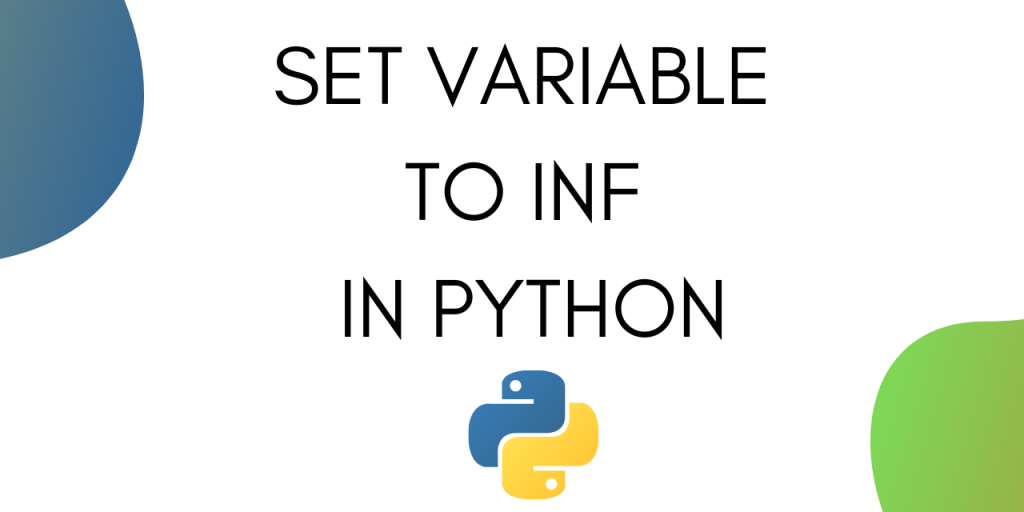# Infinity in Python – Set a Python Variable Value to InfinityA simple number cannot represent your dataset? How about setting your variable value to infinity in Python? Today we’re talking about just that!

While coding in Python, we often need to initialize a variable with a large positive or large negative value. This is very common when comparing variables to calculate the minimum or maximum in a set.

Positive infinity in Python is considered to be the largest positive value and negative infinity is considered to be the largest negative number.

In this tutorial, we will learn three ways to initialize variables with positive and negative infinity. Along with that, we will also learn how to check whether a variable is infinity or not and also perform some arithmetic operations on these variables.

Let’s get started.

## Initializing Float Variables with Infinity in Python

The most simple way to set a variable to positive or negative infinity without using any modules is by using float.

You can set a variable to positive infinity by using the following line of code:

```p_inf = float('inf')
```

To print the value of the variable use :

```print('Positive Infinity = ',p_inf)
```

Output :

```Positive Infinity =  inf
```

To initialize a variable with negative infinity use :

```n_inf = float('-inf')
print('Negative Infinity = ',n_inf)
```

Output :

```Negative Infinity =  -inf
```

## Initializing Variables with infinity Using Numpy module

You can also use the popular Numpy module to initialize variables with positive or negative infinity.

Let’s start by importing the Numpy module.

```import Numpy as np
```

Now we can use the module to initialize a variable to positive infinity as shown below :

```p_inf = np.inf
print('Positive Infinity = ',p_inf)
```

The output comes out as :

```Positive Infinity =  inf
```

To initialize a variable with negative infinity use :

```n_inf = -np.inf
print('Negative Infinity = ',n_inf)
```

The output comes out as :

```Negative Infinity =  -inf
```

### Complete code

The complete code from this section is given below.

```import Numpy as np

#positive inf
p_inf = np.inf
print('Positive Infinity = ',p_inf)

#negative inf
n_inf = -np.inf
print('Negative Infinity = ',n_inf)
```

## Initializing Variables with Infinity in Python Using Math module

The third method for initializing variables to infinity is by using the math module in python.

Let’s start by importing the module.

```import math
```

To set a variable to positive infinity using Math module use the following line of code :

```p_inf = math.inf
print('Positive Infinity = ',p_inf)
```

Output :

```Positive Infinity =  inf
```

To set a variable to negative infinity using Math module use the following line of code :

```n_inf = -math.inf
print('Negative Infinity = ',n_inf)
```

Output :

```Negative Infinity =  -inf
```

Apart from this, the Math module also gives you a method that lets you check if a variable is set to infinity or not.

You can check it using the following line of code :

```math.isinf(p_inf)
```

Output :

```True
```
```math.isinf(n_inf)
```

Output :

```True
```

### Complete Code

The complete code from this section is given below :

```import math

#positive inf
p_inf = math.inf
print('Positive Infinity = ',p_inf)

#negative inf
n_inf = -math.inf
print('Negative Infinity = ',n_inf)

#check
print(math.isinf(p_inf))
print(math.isinf(n_inf))

```

## Arithmetic Operations on infinity in Python

Let’s try doing some arithmetic operations with the variables set as positive and negative infinity.

### 1. Addition Operation on Infinity Values

Let’s see what happens when we add a number to positive infinity and negative infinity.

```a = p_inf + 100
print(a)
```

Output :

```inf
```
```a = n_inf + 100
print(a)
```

Output :

```-inf
```

### 2. Subtraction on Infinity Values

Let’s try subtracting a number from positive and negative infinity.

```a = p_inf - 100
print(a)
```

Output :

```inf
```
```a = n_inf - 100
print(a)
```

Output :

```-inf
```

We can see that adding or subtracting a value to and from infinity doesn’t have any impact.

Let’s see what happens when we perform arithmetic operations between two infinities.

### 3. Arithmetic Operations between two infinities

Let’s try adding positive and negative infinity and see the result.

```a = p_inf + n_inf
print(a)
```

Output :

```nan
```

We get the output as nan, which is short for not a number. Therefore we can say that this operation is not well defined. To know more about nan, read this tutorial.

Let’s see what happens when we multiply positive and negative infinity.

```a = p_inf * n_inf
print(a)
```

Output :

```-inf
```

We get the negative infinity as the magnitudes get multiplied and the sign is negative because of negative infinity.

Let’s see what happens when we perform division between positive and negative infinity.

```a = p_inf / n_inf
print(a)
```

Output :

```nan
```

Division of infinity with infinity is again not defined hence we get nan.

## Conclusion

This tutorial was about different ways you can use to initialize variables with positive and negative infinity. We also covered some arithmetic operations involving positive and negative infinity.